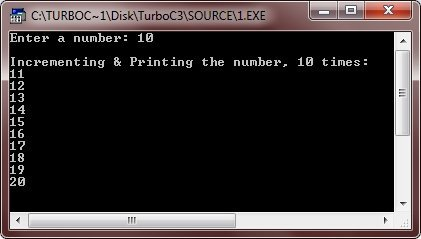# C++ Loops

Loop allows us to execute a block of code or statement(s) several number of times. C++ provides the following types of loop to handle the looping requirements in program. The detailed description of these loops are provided in C++ for while do-while Loop tutorial.

The detail description of all the loops provided in the above table, is discussed in the next tutorial. For now, let's take some example on looping. Let's start with for loop program.

```/* C++ Loops Example */

#include<iostream.h>
#include<conio.h>
void main()
{
clrscr();
int num, l;
cout<<"Enter a number: ";
cin>>num;
cout<<"\nIncrementing & Printing the number, 10 times:\n";
for(l=0; l<10; l++)
{
num++;
cout<<num<<"\n";
}
getch();
}```

Here is the sample run of the above C++ programHere is an example program demonstrating while loop in C++

```/* C++ Loops Program */

#include<iostream.h>
#include<conio.h>
void main()
{
clrscr();
int num, l=0;
cout<<"Enter a number: ";
cin>>num;
cout<<"\nIncrementing & Printing the number, 10 times:\n";
while(l<10)
{
num++;
cout<<num<<"\n";
l++;
}
getch();
}```

Here is the sample run of this C++ program, producing the same result as aboveHere is an example program, demonstrating do-while loop in C++

```/* C++ Loops Example */

#include<iostream.h>
#include<conio.h>
void main()
{
clrscr();
int num, l=0;
cout<<"Enter a number: ";
cin>>num;
cout<<"\nIncrementing & Printing the number, 10 times:\n";
do
{
num++;
cout<<num<<"\n";
l++;
}while(l<10);
getch();
}```

Here is the sample run of the above C++ program, also producing the same output as the above two programs producedYou will learn all about loop (one by one) in detail in next chapter.

### More Examples

Here are some more examples listed, that you may like:

Tools
Calculator

Quick Links
Signup - Login - Give Online Test